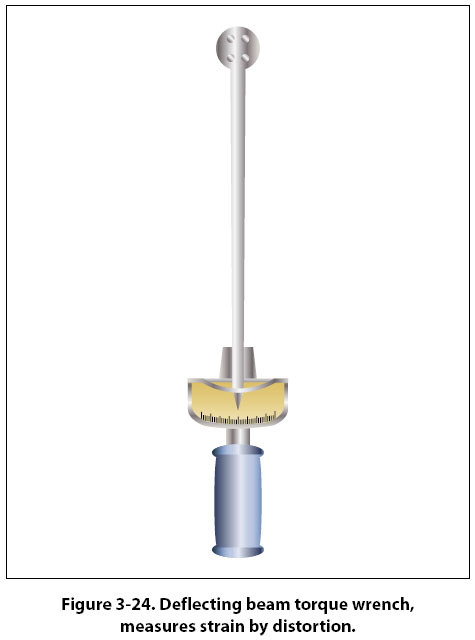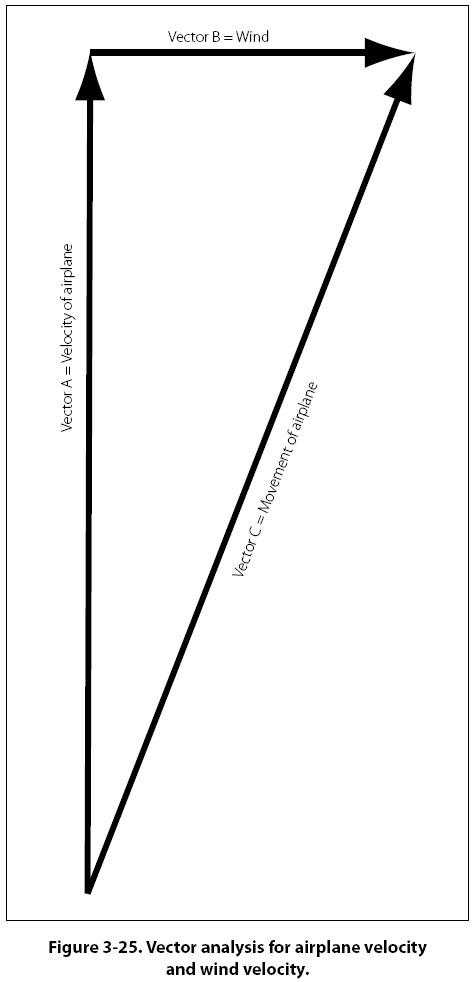Strain If the stress acting on an object is great enough, it can cause the object to change its shape or to become distorted. One characteristic of matter is that it tends to be elastic, meaning it can be forced out of shape when a force is applied, and then return to its original shape when the force is removed. When an object becomes distorted by an applied force, the object is said to be strained. On turbine engine test cells, the thrust of the engine is typically measured by what are called strain gages. When the force (thrust) of the engine is pulling out against the strain gages, the amount of distortion is measured and then translated into the appropriate thrust reading. A deflecting beam style of torque wrench uses the strain on the drive end of the wrench and the resulting distortion of the beam to indicate the amount of torque on a bolt or nut. [Figure 3-24]Motion The study of the relationship between the motion of bodies or objects and the forces acting on them is often called the study of “force and motion." In a more specific sense, the relationship between velocity, acceleration, and distance is known as kinematics. Uniform Motion Motion may be defined as a continuing change of position or place, or as the process in which a body undergoes displacement. When an object is at different points in space at different times, that object is said to be in motion, and if the distance the object moves remains the same for a given period of time, the motion may be described as uniform. Thus, an object in uniform motion always has a constant speed. Speed and Velocity In everyday conversation, speed and velocity are often used as if they mean the same thing. In physics they have definite and distinct meanings. Speed refers to how fast an object is moving, or how far the object will travel in a specific time. The speed of an object tells nothing about the direction an object is moving. For example, if the information is supplied that an airplane leaves New York City and travels 8 hours at a speed of 150 mph, this information tells nothing about the direction in which the airplane is moving. At the end of 8 hours, it might be in Kansas City, or if it traveled in a circular route, it could be back in New York City. Velocity is that quantity in physics which denotes both the speed of an object and the direction in which the object moves. Velocity can be defined as the rate of motion in a particular direction. Velocity is also described as being a vector quantity, a vector being a line of specific length, having an arrow on one end or the other. The length of the line indicates the number value and the arrow indicates the direction in which that number is acting. Two velocity vectors, such as one representing the velocity of an airplane and one representing the velocity of the wind, can be added together in what is called vector analysis. Figure 3-25 demonstrates this, with vectors “A" and “B" representing the velocity of the airplane and the wind, and vector “C" being the resultant. With no wind, the speed and direction of the airplane would be that shown by vector “A." When accounting for the wind direction and speed, the airplane ends up flying at the speed and direction shown by vector “C."Imagine that an airplane is flying in a circular pattern at a constant speed. Because of the circular pattern, the airplane is constantly changing direction, which means the airplane is constantly changing velocity. The reason for this is the fact that velocity includes direction. To calculate the speed of an object, the distance it travels is divided by the elapsed time. If the distance is measured in miles and the time in hours, the units of speed will be miles per hour (mph). If the distance is measured in feet and the time in seconds, the units of speed will be feet per second (fps). To convert mph to fps, multiply by 1.467. Velocity is calculated the same way, the only difference being it must be recalculated every time the direction changes.
 ©AvStop Online Magazine                                                                                                                                                      Contact Us              Return To Books